Tangent Additions and the Pascal Triangle

# An Approach to Mathematic Functions Basics

## Section XLIII - Tangent Additions and the Pascal Triangle

### Summary for the Section :

• In this Section , we shall attempt to show that :

• for the [ fraction ] expressing the { tangent of [ N*Theta ] } in terms of the { tangent of [ Theta ] } ,

• the co-efficients in the Nominator and the co-efficients in the Denominator

• are always members of the same row of the Pascal Triangle .

And we shall also create the { 4-Colors Pascal Triangle } , as per the diagram on-the-right below , for our use in the next Section .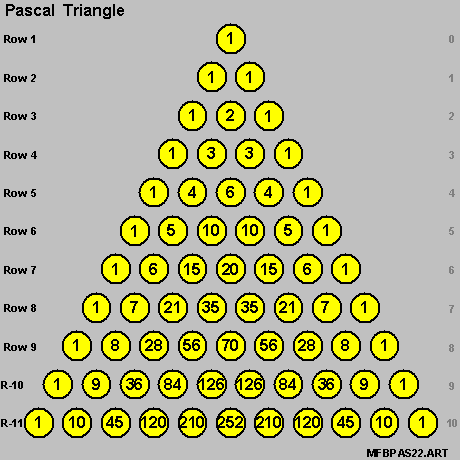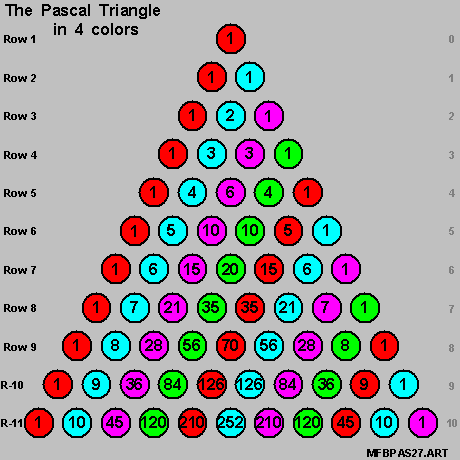### Doing a Quick Example with the { Tangent of [ 8*Theta ] } :

• Let us now quickly bring-in the formula for the { Tangent of [ 8*Theta ] } :

•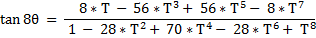where :

•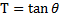And we bring-in also this set of 2 diagrams below for a quick comparison .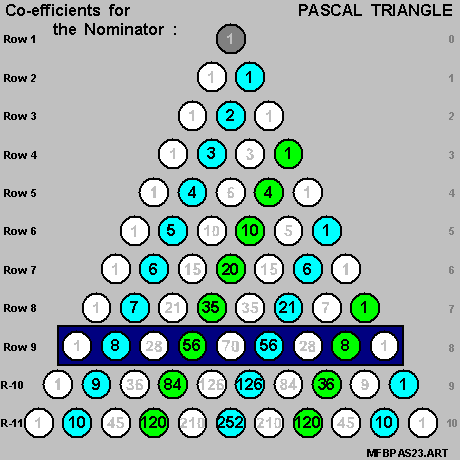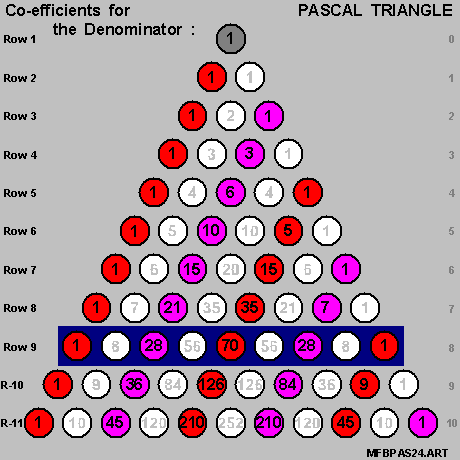And we see here that :

• FIRST :

• The co-efficients in the Nominator , namely [ +8 / -56 / +56 / -8 ] ,

• are members of [ Row 9 ] of the Pascal Triangle ,

• as marked-off in [ cyan-color ] and [ green-color ] in the diagram on-the-left above .

• SECOND :

• The co-efficients in the Denominator , namely [ +1 / -28 / +70 / -28 / +1 ] ,

• are members of [ Row 9 ] of the Pascal Triangle ,

• as marked-off in [ red-color ] and [ magenta color ] in the diagram on-the-right above .

• Let us now make a general statement on the pattern of these co-efficients , next .

### Re-counting the Co-efficients :

• Let us bring-in again the results of our calculation process in the last Section and we have :

•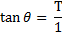•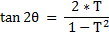•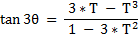•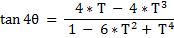•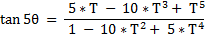•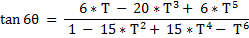•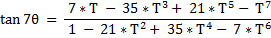•And we bring in also this set of 2 diagrams on the Pascal Triangle for our demonstration purposes :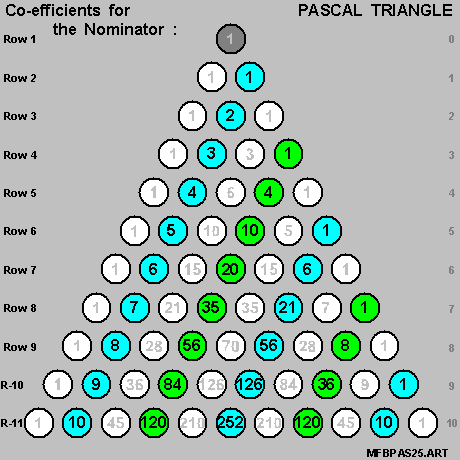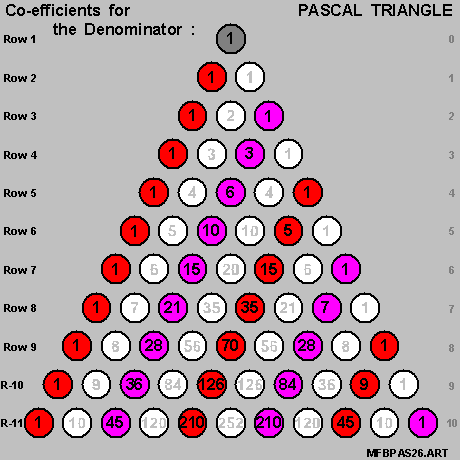And we see here that :

• for the [ fraction ] expressing the { Tangent of [ N*Theta ] } in terms of the { Tangent of [ Theta ] } :

FIRST :

• The co-efficients in the Nominator and the Denominator are members of [ Row N+1 ] of the Pascal Triangle .

SECOND :

• The co-efficients in the Nominator are as marked-off [ cyan-color ] and [ green-color ] in the diagram on-the-left above ,

with :

• [ cyna-color ] members spotting [ positive signs ] and [ green-color ] members spotting [ negative signs ] .

THIRD :

• The co-efficients in the Denominator are as marked-off [ red-color ] and [ magenta-color ] in the diagram on-the-right above ,

with :

• [ red-color ] members spotting [ positive signs ] and [ magenta-color ] members spotting [ negative signs ] .

### Creating the { 4-Colors Pascal Triangle } :

• Let us now consolidate the 2 diagrams above into single diagram ,

• to create the { 4-Colors Pascal Triangle } ,

• as the diagram on-the-right below .• This is an interesting diagram which we shall put into good use in the next Section .